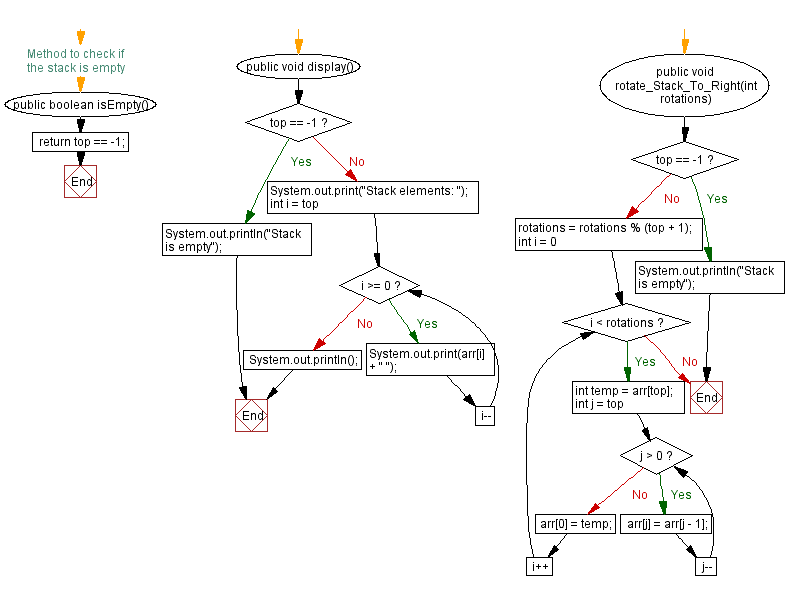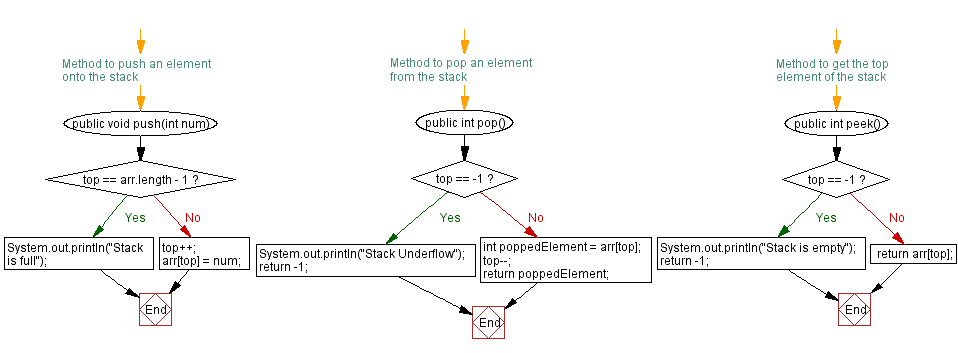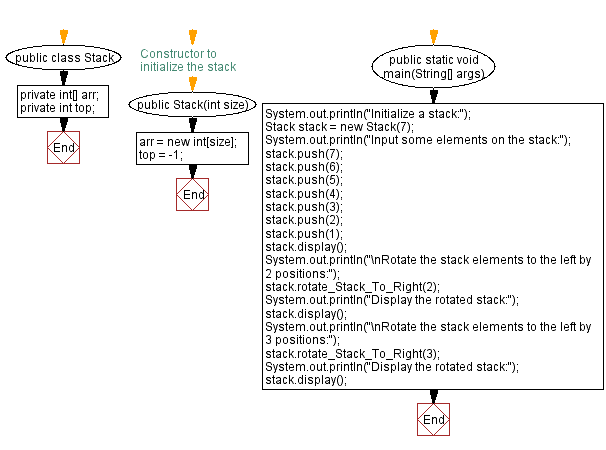# Java Exercises: Rotate the stack elements to the left

## Java Stack: Exercise-12 with Solution

Write a Java program to rotate the stack elements to the left direction.

Sample Solution:

Java Code:

``````import java.util.Scanner;
public class Stack {
private int[] arr;
private int top;

// Constructor to initialize the stack
public Stack(int size) {
arr = new int[size];
top = -1;
}

// Method to push an element onto the stack
public void push(int num) {
if (top == arr.length - 1) {
System.out.println("Stack is full");
} else {
top++;
arr[top] = num;
}
}

// Method to pop an element from the stack
public int pop() {
if (top == -1) {
System.out.println("Stack Underflow");
return -1;
} else {
int poppedElement = arr[top];
top--;
return poppedElement;
}
}

// Method to get the top element of the stack
public int peek() {
if (top == -1) {
System.out.println("Stack is empty");
return -1;
} else {
return arr[top];
}
}

// Method to check if the stack is empty
public boolean isEmpty() {
}

public void display() {
if (top == -1) {
System.out.println("Stack is empty");
} else {
System.out.print("Stack elements: ");
for (int i = top; i >= 0; i--) {
System.out.print(arr[i] + " ");
}
System.out.println();
}
}

public void rotate_Stack_To_Right(int rotations) {
if (top == -1) {
System.out.println("Stack is empty");
return;
}
rotations = rotations % (top + 1);
for (int i = 0; i < rotations; i++) {
int temp = arr[top];
for (int j = top; j > 0; j--) {
arr[j] = arr[j - 1];
}
arr = temp;
}
}

public static void main(String[] args) {
System.out.println("Initialize a stack:");
Stack stack = new Stack(7);
System.out.println("Input some elements on the stack:");
stack.push(7);
stack.push(6);
stack.push(5);
stack.push(4);
stack.push(3);
stack.push(2);
stack.push(1);
stack.display();
System.out.println("\nRotate the stack elements to the left by 2 positions:");
stack.rotate_Stack_To_Right(2);
System.out.println("Display the rotated stack:");
stack.display();
System.out.println("\nRotate the stack elements to the left by 3 positions:");
stack.rotate_Stack_To_Right(3);
System.out.println("Display the rotated stack:");
stack.display();

}
}
```
```

Sample Output:

```Initialize a stack:
Input some elements on the stack:
Stack elements: 1 2 3 4 5 6 7

Rotate the stack elements to the left by 2 positions:
Display the rotated stack:
Stack elements: 3 4 5 6 7 1 2

Rotate the stack elements to the left by 3 positions:
Display the rotated stack:
Stack elements: 6 7 1 2 3 4 5

```

Flowchart:Live Demo:

Java Code Editor:

Improve this sample solution and post your code through Disqus

Java Stack Previous: Rotate the stack elements to the right.
Java Stack Exercises Next: Remove a specific element from a stack.

What is the difficulty level of this exercise?

Test your Programming skills with w3resource's quiz.

﻿